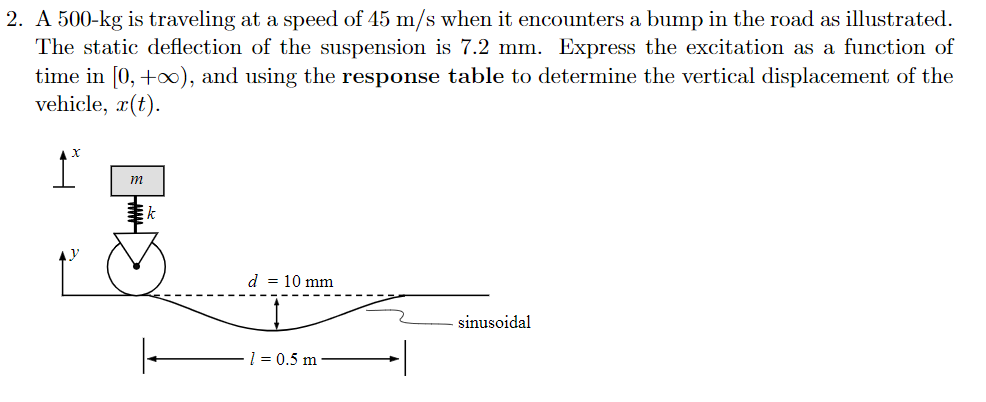# 2. A 500-kg is traveling at a speed of 45 m/s when it encounters a bump in the road as illustratedThe static deflection of the suspension is 7.2 mm. Express the excitation as a function oftime in [0, +oo), and using the response table to determine the vertical displacement of thevehicle, rt)d 10 mmsinusoidall 0.5 m

Question
15 views

. A 500-kg is traveling at a speed of 45 m/s when it encounters a bump in the road as illustrated. The static deflection of the suspension is 7.2 mm. Express the excitation as a function of time in [0;+infinity), and using the response table to determine the vertical displacement of the vehicle,x(t)help_outlineImage Transcriptionclose2. A 500-kg is traveling at a speed of 45 m/s when it encounters a bump in the road as illustrated The static deflection of the suspension is 7.2 mm. Express the excitation as a function of time in [0, +oo), and using the response table to determine the vertical displacement of the vehicle, rt) d 10 mm sinusoidal l 0.5 m fullscreen
check_circle

Step 1

Given data:

The mass of the object = 500 kg

Speed = 45 m/s

The static deflection of the suspension = 7.2 mm

Step 2

Calculate the stiffness of the spring.

Step 3

Calculate the angu...

### Want to see the full answer?

See Solution

#### Want to see this answer and more?

Solutions are written by subject experts who are available 24/7. Questions are typically answered within 1 hour.*

See Solution
*Response times may vary by subject and question.
Tagged in

### Mechanical Vibrations